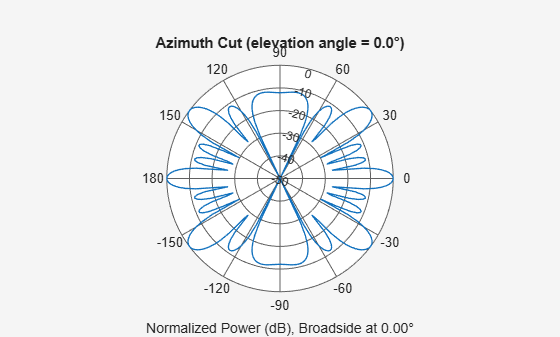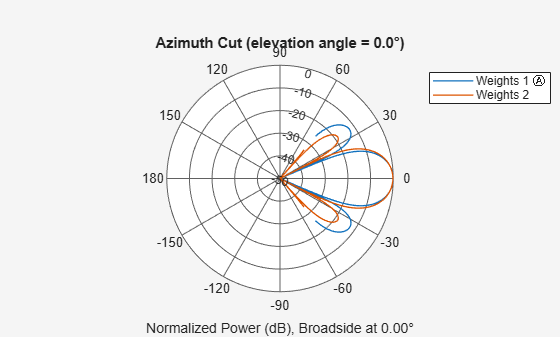# plotResponse

System object: phased.PartitionedArray
Package: phased

Plot response pattern of array

## Syntax

```plotResponse(H,FREQ,V) plotResponse(H,FREQ,V,Name,Value) hPlot = plotResponse(___) ```

## Description

`plotResponse(H,FREQ,V)` plots the array response pattern along the azimuth cut, where the elevation angle is 0. The operating frequency is specified in `FREQ`. The propagation speed is specified in `V`.

`plotResponse(H,FREQ,V,Name,Value)` plots the array response with additional options specified by one or more `Name,Value` pair arguments.

`hPlot = plotResponse(___)` returns handles of the lines or surface in the figure window, using any of the input arguments in the previous syntaxes.

## Input Arguments

 `H` Array object. `FREQ` Operating frequency in hertz. Typical values are within the range specified by a property of `H.Array.Element`. That property is named `FrequencyRange` or `FrequencyVector`, depending on the type of element in the array. The element has zero response at frequencies outside that range. If `FREQ` is a nonscalar row vector, the plot shows multiple frequency responses on the same axes. `V` Propagation speed in meters per second.

### Name-Value Arguments

Specify optional pairs of arguments as `Name1=Value1,...,NameN=ValueN`, where `Name` is the argument name and `Value` is the corresponding value. Name-value arguments must appear after other arguments, but the order of the pairs does not matter.

Before R2021a, use commas to separate each name and value, and enclose `Name` in quotes.

`CutAngle`

Cut angle specified as a scalar. This argument is applicable only when `RespCut` is `'Az'` or `'El'`. If `RespCut` is `'Az'`, `CutAngle` must be between –90 and 90. If `RespCut` is `'El'`, `CutAngle` must be between –180 and 180.

Default: `0`

`Format`

Format of the plot, using one of `'Line'`, `'Polar'`, or `'UV'`. If you set `Format` to `'UV'`, `FREQ` must be a scalar.

Default: `'Line'`

`NormalizeResponse`

Set this value to `true` to normalize the response pattern. Set this value to `false` to plot the response pattern without normalizing it. This parameter is not applicable when you set the `Unit` parameter value to `'dbi'`.

Default: `true`

`OverlayFreq`

Set this value to `true` to overlay pattern cuts in a 2-D line plot. Set this value to `false` to plot pattern cuts against frequency in a 3-D waterfall plot. If this value is `false`, then `FREQ` must be a vector with at least two entries.

This parameter applies only when `Format` is not `'Polar'` and `RespCut` is not `'3D'`.

Default: `true`

`Polarization`

Specify the polarization options for plotting the array response pattern. The allowable values are ```|'None' | 'Combined' | 'H' | 'V' |``` where:

• `'None'` specifies plotting a nonpolarized response pattern

• `'Combined'` specifies plotting a combined polarization response pattern

• `'H'` specifies plotting the horizontal polarization response pattern

• `'V'` specifies plotting the vertical polarization response pattern

For arrays that do not support polarization, the only allowed value is `'None'`. This parameter is not applicable when you set the `Unit` parameter value to `'dbi'`.

Default: `'None'`

`RespCut`

Cut of the response. Valid values depend on `Format`, as follows:

• If `Format` is `'Line'` or `'Polar'`, the valid values of `RespCut` are `'Az'`, `'El'`, and `'3D'`. The default is `'Az'`.

• If `Format` is `'UV'`, the valid values of `RespCut` are `'U'` and `'3D'`. The default is `'U'`.

If you set `RespCut` to `'3D'`, `FREQ` must be a scalar.

`SteerAng`

Subarray steering angle. `SteerAng` can be either a 2-element column vector or a scalar.

If `SteerAng` is a 2-element column vector, it has the form [azimuth; elevation]. The azimuth angle must be between –180 and 180 degrees, inclusive. The elevation angle must be between –90 and 90 degrees, inclusive.

If `SteerAng` is a scalar, it specifies the azimuth angle. In this case, the elevation angle is assumed to be 0.

This option is applicable only if the `SubarraySteering` property of `H` is `'Phase'` or `'Time'`.

Default: `[0;0]`

`Unit`

The unit of the plot. Valid values are `'db'`, `'mag'`, `'pow'`, or `'dbi'`. This parameter determines the type of plot that is produced.

Unit valuePlot type
`db`power pattern in dB scale
`mag`field pattern
`pow`power pattern
`dbi`directivity

Default: `'db'`

`Weights`

Weight values applied to the array, specified as a length-N column vector or N-by-M matrix. The dimension N is the number of subarrays in the array. The interpretation of M depends upon whether the input argument `FREQ` is a scalar or row vector.

Weights DimensionFREQ DimensionPurpose
N-by-1 column vectorScalar or 1-by-M row vectorApply one set of weights for the same single frequency or all M frequencies.
N-by-M matrixScalarApply all of the M different columns in `Weights` for the same single frequency.
1-by-M row vectorApply each of the M different columns in `Weights` for the corresponding frequency in `FREQ`.

`AzimuthAngles`

Azimuth angles for plotting subarray response, specified as a row vector. The `AzimuthAngles` parameter sets the display range and resolution of azimuth angles for visualizing the radiation pattern. This parameter is allowed only when the `RespCut` parameter is set to `'Az'` or `'3D'` and the `Format` parameter is set to `'Line'` or `'Polar'`. The values of azimuth angles should lie between –180° and 180° and must be in nondecreasing order. When you set the `RespCut` parameter to `'3D'`, you can set the `AzimuthAngles` and `ElevationAngles` parameters simultaneously.

Default: `[-180:180]`

`ElevationAngles`

Elevation angles for plotting subarray response, specified as a row vector. The `ElevationAngles` parameter sets the display range and resolution of elevation angles for visualizing the radiation pattern. This parameter is allowed only when the `RespCut` parameter is set to `'El'` or `'3D'` and the `Format` parameter is set to `'Line'` or `'Polar'`. The values of elevation angles should lie between –90° and 90° and must be in nondecreasing order. When you set the `RespCut` parameter to `'3D'`, you can set the `ElevationAngles` and `AzimuthAngles` parameters simultaneously.

Default: `[-90:90]`

`UGrid`

U coordinate values for plotting subarray response, specified as a row vector. The `UGrid` parameter sets the display range and resolution of the U coordinates for visualizing the radiation pattern in U/V space. This parameter is allowed only when the `Format` parameter is set to `'UV'` and the `RespCut` parameter is set to `'U'` or `'3D'`. The values of `UGrid` should be between –1 and 1 and should be specified in nondecreasing order. You can set the `UGrid` and `VGrid` parameters simultaneously.

Default: `[-1:0.01:1]`

`VGrid`

V coordinate values for plotting subarray response, specified as a row vector. The `VGrid` parameter sets the display range and resolution of the V coordinates for visualizing the radiation pattern in U/V space. This parameter is allowed only when the `Format` parameter is set to `'UV'` and the `RespCut` parameter is set to `'3D'`. The values of `VGrid` should be between –1 and 1 and should be specified in nondecreasing order. You can set the `VGrid` and `UGrid` parameters simultaneously.

Default: `[-1:0.01:1]`

## Examples

expand all

Plot the azimuth response of a 4-element ULA partitioned into two 2-element ULA's. The element spacing is one-half wavelength.

Create the ULA, and partition it into two 2-element ULA's.

```sULA = phased.ULA('NumElements',4,'ElementSpacing',0.5); sPA = phased.PartitionedArray('Array',sULA,... 'SubarraySelection',[1 1 0 0;0 0 1 1]);```

Plot the azimuth response of the array. Assume the operating frequency is 1 GHz and the propagation speed is the speed of light.

```fc = 1e9; pattern(sPA,fc,[-180:180],0,'Type','powerdb',... 'CoordinateSystem','polar',... 'Normalize',true)```Convert a 2-by-6 URA of isotropic antenna elements into a 1-by-3 partitioned array so that each subarray of the partitioned array is a 2-by-2 URA. Assume that the frequency response of the elements lies between 1 and 6 GHz. The elements are spaced one-half wavelength apart corresponding to the highest frequency of the element response. Plot an azimuth cut from -50 to 50 degrees for two different sets of weights. For partitioned arrays, weights are applied to the subarrays instead of the elements.

Set up the partitioned array.

```fmin = 1e9; fmax = 6e9; c = physconst('LightSpeed'); lam = c/fmax; s_iso = phased.IsotropicAntennaElement(... 'FrequencyRange',[fmin,fmax],... 'BackBaffled',false); s_ura = phased.URA('Element',s_iso,'Size',[2,6],... 'ElementSpacing',[lam/2,lam/2]); subarraymap = [[1,1,1,1,0,0,0,0,0,0,0,0];... [0,0,0,0,1,1,1,1,0,0,0,0];... [0,0,0,0,0,0,0,0,1,1,1,1]]; s_pa = phased.PartitionedArray('Array',s_ura,... 'SubarraySelection',subarraymap);```

Plot the response of the array at 5 GHz over the restricted range of azimuth angles.

```fc = 5e9; wts = [[1,1,1]',[.862,1.23,.862]']; plotResponse(s_pa,fc,c,'RespCut','Az',... 'AzimuthAngles',[-50:0.1:50],... 'Unit','db','Format','Polar',... 'Weights',wts);```The plot of the response shows the broadening of the main lobe and the reduction of the strength of the sidelobes caused by the weight tapering.

Next, plot an azimuth cut of the directivity of the array at 5 GHz over the restricted range of azimuth angles for the two different sets of weights.

```fc = 5e9; wts = [[1,1,1]',[.862,1.23,.862]']; plotResponse(s_pa,fc,c,'RespCut','Az',... 'AzimuthAngles',[-50:0.1:50],... 'Unit','dbi',... 'Weights',wts);```